# What is an Oscillator ? What do you understand by damped and undamped oscillations ? Explain the operation of a tank circuit.

## Sinusoidal Oscillator

An electronic device that generates sinusoidal oscillations of desired frequency is known as a sinusoidal oscillator.

The oscillator does not create energy, but it acts as an energy converter. It receives d.c. energy and changes it into a.c. energy of desired frequency. The frequency of the oscillations depends upon the constants of the device.

Although oscillations can be produced by mechanical devices such as alternators, but electronic oscillators have the following advantages:

1. An oscillator is a non-rotating device. Hence, there is little wear and tear and hence longer life.
2. Due to the absence of moving parts, the operation of an oscillator is quite silent.
3. An oscillator can produce waves from small i.e. 20 Hz to extremely high frequencies i.e.>100 MHz.
4. The frequency of oscillations can be easily changed when desired.
5. It has good frequency stability i.e. frequency once set remains constant for a considerable period of time.
6. It has very high efficiency.

### Types of Sinusoidal Oscillations

Sinusoidal oscillations can be of two types, damped oscillations and undamped oscillations.

#### Damped Oscillations :

The electrical oscillations whose amplitude goes on decreasing with time are called damped oscillations. Fig.1(i) shows waveform of damped electrical oscillations.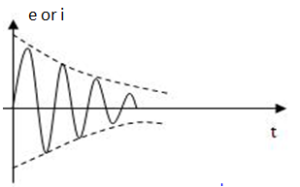Fig.1(i)

The oscillator which generates these oscillations, losses some energy during each oscillation. As no means are provided to compensate for these losses,hence the amplitude of the generated wave decreases gradually.

It can be noted that the frequency of oscillations remains unchanged since it depends upon the constant of the electrical system.

#### Undamped Oscillations :

The electrical oscillations whose amplitude remains constant with time are called undamped oscillations. Fig.1(ii) shows waveform of undamped electrical oscillations.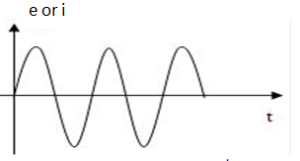Fig.1(ii)

Although the electrical system in which these oscillations are being generated has also losses, but now right amount of energy is being supplied to overcome the losses. Hence, the amplitude of the generated wave remains constant.

An oscillator is mainly required to produce undamped oscillations for utilizing in various electronics equipment.

### Oscillatory Circuit

A circuit which produces electrical oscillations of any desired frequency is known as oscillatory circuit or tank circuit.

A simple oscillatory circuit consists of a capacitor (C) and inductance coil (L) in parallel as shown in fig.2(i).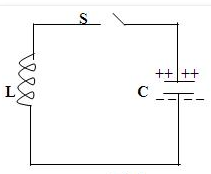Fig.2(i)

This electrical system can produce electrical oscillations of frequency determined by the values of L and C.

(i) Now suppose the capacitor is charged from a d.c. source with a polarity as shown in fig.2(i). In this position, the upper plate of capacitor has deficit of electrons and the lower plate has excess of electrons. Therefore, there is a voltage across the capacitor and the capacitor has electrostatic energy.

(ii) When switch S is closed as shown in fig.2(ii), the capacitor will discharge through inductance and the electron will flow in the direction indicated by the arrow. This current flow sets up magnetic field around the coil. Due to the inductive effect, the current builds up slowly towards a maximum value. The circuit current will be maximum when the capacitor is fully discharged. At this instant, electrostatic energy is zero but because of maximum current the magnetic field energy around the coil is maximum. So the electrostatic energy across the capacitor is completely converted to magnetic field energy around the coil.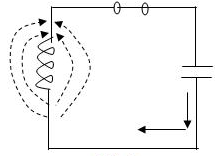Fig.2(ii)

(iii) Once the capacitor is discharged, the magnetic field will begin to collapse and produce a counter e.m.f. This counter e.m.f will keep the current flowing in the same direction. The result is that the capacitor is now charged with opposite polarity, making upper plate of capacitor negative and lower plate positive as shown in fig.2(iii).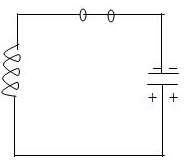Fig.2(iii)

(iv) After the collapsing field has recharged the capacitor, the capacitor now begins to discharge; current now flowing in the opposite direction. fig.2(iv) shows capacitor fully discharged and maximum current flowing.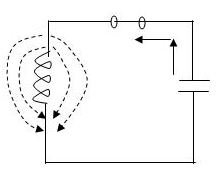Fig.2(iv)

The sequence of charge and discharge results in alternating motion of electrons or an oscillating current.

The energy is alternately stored in the electric field of the capacitor C and the magnetic field of inductance coil L.

This interchange of energy between L and C is repeated over and again resulting in the production of oscillations.

Waveform:

In a practical tank circuit there are resistive and radiation losses in the coil and dielectric losses in the capacitor. During each cycle, a small part of the originally imparted energy is used up to overcome these losses. The result is that the amplitude of oscillating current decreases gradually and eventually it becomes zero. Therefore, the tank circuit by itself will produce damped oscillations as shown in fig.3.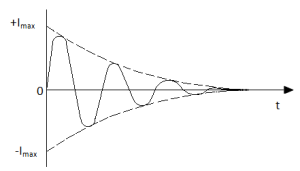Fig.3

Frequency of Oscillations:

The frequency of oscillations in the tank circuit is the resonant frequency given by: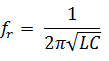### Undamped Oscillations from Tank Circuit

In order to make the oscillations in the tank circuit undamped, it is necessary to supply correct amount of energy to the tank circuit at the proper time intervals to meet the losses.

Thus any energy which would be applied to the circuit must have a polarity conforming to the existing polarity  at the instant of application of energy.

If the applied energy is of opposite polarity, it would oppose the energy in the tank circuit, causing stoppage of oscillations.

Therefore, in order to make the oscillations in the tank circuit undamped, the following conditions must be fulfilled:

1. The amount of energy supplied should be sufficient to meet the losses in the tank circuit and the a.c. energy removed from the circuit by the load.For example, if losses in LC circuit equals to 5 mW and a.c. output being taken is 100 mW, then power of 105 mW should be continuously supplied to the circuit.
2. The applied energy should have the same frequency as that of the oscillations in the tank circuit.
3. The applied energy should be in phase with the oscillations set up in the tank circuit i.e. it should aid the tank circuit oscillations.

If the conditions are fulfilled, the circuit will produce continuous undamped output as shown in fig.4.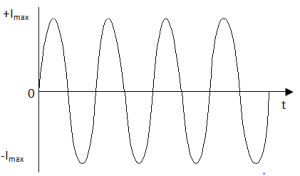Fig.4